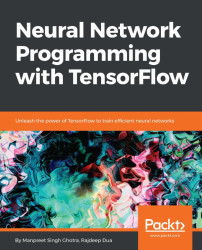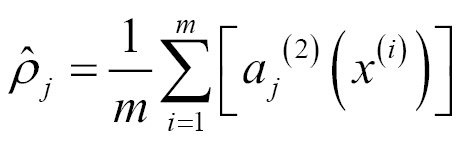•#### Neural Network Programming with Tensorflow#### Overview of this book

If you're aware of the buzz surrounding the terms such as "machine learning," "artificial intelligence," or "deep learning," you might know what neural networks are. Ever wondered how they help in solving complex computational problem efficiently, or how to train efficient neural networks? This book will teach you just that. You will start by getting a quick overview of the popular TensorFlow library and how it is used to train different neural networks. You will get a thorough understanding of the fundamentals and basic math for neural networks and why TensorFlow is a popular choice Then, you will proceed to implement a simple feed forward neural network. Next you will master optimization techniques and algorithms for neural networks using TensorFlow. Further, you will learn to implement some more complex types of neural networks such as convolutional neural networks, recurrent neural networks, and Deep Belief Networks. In the course of the book, you will be working on real-world datasets to get a hands-on understanding of neural network programming. You will also get to train generative models and will learn the applications of autoencoders. By the end of this book, you will have a fair understanding of how you can leverage the power of TensorFlow to train neural networks of varying complexities, without any hassle. While you are learning about various neural network implementations you will learn the underlying mathematics and linear algebra and how they map to the appropriate TensorFlow constructs.
Title PageCreditswww.PacktPub.comCustomer FeedbackPrefaceFree Chapter
Maths for Neural NetworksDeep Feedforward NetworksOptimization for Neural NetworksConvolutional Neural NetworksRecurrent Neural NetworksGenerative ModelsDeep Belief NetworkingAutoencodersResearch in Neural NetworksGetting started with TensorFlow## Sparse autoencoder

In this section, we will look at how adding sparsity to the cost function helps in reducing the cost of training. Most of the code remains the same, but the primary changes are in the way the cost function is calculated.

### KL divergence

Let's first try to understand KL divergence, which is used to add sparsity to the cost function.

We can think of a neuron as active (or firing) if a neuron's output value is close to one, and inactive if its output value is close to zero. We would like to constrain the neurons to be inactive most of the time. This discussion assumes a sigmoid activation function. Recall that a(2)j denotes the activation of the hidden unit j in the autoencoder. This notation does not state explicitly what the input was that led to this activation. We will write a(2)j(x) to denote the activation of the hidden unit when the network is given a specific input x. Further, letbe the average activation of the hidden unit j (averaged over the training set). We would...